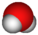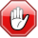2022: SklogWiki celebrates 15 years on-line

# TIP4P/Ice model of waterThis article is a 'stub' about water and/or ice. It has no, or next to no, content. It is here at the moment to help form part of the structure of SklogWiki. If you add material to this article, remove the {{Stub-water}} template from this page.This page contains numerical values and/or equations. If you intend to use ANY of the numbers or equations found in SklogWiki in any way, you MUST take them from the original published article or book, and cite the relevant source accordingly.$r_{\mathrm {OH}}$ (Å)$\angle$HOH , deg$\sigma$ (Å)$\epsilon/k$ (K) q(O) (e) q(H) (e) q(M) (e)$r_{\mathrm {OM}}$ (Å) 0.9572 104.52 3.1668 106.1 0 0.5897 -2q(H) 0.1577